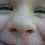## Beautiful Running LED Animated Demo : Circuit using 555 in Astable Mode

by realfinetime  |  in Running LED Circuit at  02:49

Important : If there is any difficulty in the working of circuit, reduce the value of R2 and try the circuit again. Reduce the value in steps ( 1 MOhm, 10 KOhm, 1 Kohm, 0 Ohm ).

Running LEDs is an interesting project for all beginners in electronics. A lot of methods are there to create a running LEDs. Same can achieved at desired speed by an astable multivibrator designed using 555 timer. We have already seen an Amazing Animation of Astable Mode Operation of 555 Timer with Circuit Diagram. It is better to read that blog to get a clear idea about the working of 555 in astable mode before starting this blog. Circuit is done as shown in the circuit given below.
Important : If there is any difficulty in the working of circuit, reduce the value of R2 and try the circuit again. Reduce the value in steps ( 1 MOhm, 10 KOhm, 1 Kohm, 0 Ohm ).

We have already seen the working of 555 in astable mode from Amazing Animation of Astable Mode Operation of 555 Timer with Circuit Diagram. Same principle is used here. Only difference is in the mode of connection of LEDs to the output pin ( pin 3 ) of 555.

In the previous blog, we used an LED to take the output. But in this circuit, we are using a series of LEDs. Alternate LEDs are anode and cathode controlled as shown in the circuit diagram. In anode controlled LEDs, cathode is connected to ground. Similarly, in cathode controlled LEDs, anode is connected to VCC. When the output voltage is HIGH, anode controlled LEDs will turn on and cathode controlled LEDs will turn off. When the output is LOW, anode controlled LEDs will turn off and cathode controlled LEDs will turn on. Frequency of the output pulse and hence the speed of running LEDs depends on the values of R1, R2 and C1. A capacitor bank is connected in between TRIG pin ( pin 2 ) and ground to change the value of C1 and hence the speed of running LEDs.

Capacitor bank has three 10nF capacitors connected in parallel. Two of these capacitors are controlled by two push button switches ( S1 and S2 ). When both switches are off, only one capacitor will be in circuit. Then C1 = 10nF. If S1 is pressed, one more capacitor will be included in the circuit. Then two 10nF capacitors will be in parallel. Net capacitance will be 20nF. If S2 is also pressed, other capacitor also get included in the circuit. Then three 10nF capacitors will come in parallel and the net capacitance will be 30nF.

Frequency of the rectangular pulses generated depends on the values of R1, R2 and C1.

Frequency, F = 1 / ( ln( 2 ) . C1 . ( R1+2R2 ) )

Ton   =  ln( 2 ) . C1 . ( R1 + R2 )

Toff   =  ln( 2 ) . C1 . R2

C1- Capacitance in Farad, R1 and R2 - Resistance in Ohms, F - Frequency in Hertz, Ton - Time for which output will be HIGH ( LED will be ON ), Toff - Time for which output will be LOW ( LED will be OFF )

When S1 and S2 are OFF

Only one capacitor will be included in the circuit. Then
R1 = R2 = 10 Mega Ohm = 10 * 106  Ohm
ln ( 2 ) = 0.693

Substituting these values in the equations, we get
F = 4.81 Hz, Ton = 0.1386 S, Toff =  0.0693 S

When S1 is ON and S2 is OFF

Two capacitors will be in parallel in the circuit. Then
R1 = R2 = 10 Mega Ohm = 10 * 106  Ohm
ln ( 2 ) = 0.693

Substituting these values in the equations, we get
F = 2.405 Hz, Ton = 0.2772 S, Toff =  0.1386 S

When S1 and S2 are ON

Three capacitors will be in parallel in the circuit. Then
R1 = R2 = 10 Mega Ohm = 10 * 106  Ohm
ln ( 2 ) = 0.693

Substituting these values in the equations, we get
F = 1.603 Hz, Ton = 0.4158 S, Toff =  0.2079 S

From the calculations, we can conclude that, when the value of capacitance increases, output frequency and hence speed of running LEDs decreases as shown in the animated image.

1.Hello, I built this circuit today, thank you for it. Can I increase the number of LED'S? I have project that needs 30.

2.An integrated circuit, sometimes called a chip or microchip, is a semiconductor wafer on which thousands or millions of tiny resistors, capacitors, and transistors are fabricated. how to discharge a capacitor with a screwdriver

## IMPORTANT NOTICE

All the circuits, published in this blog is only after testing and getting proper results in my private lab. When you try these circuits, you should check the supply voltage, polarity of components, presence of childrens nearby and shorts in the circuits. This website will not be responsible for any harm happened to you or your components caused by your carelessness.